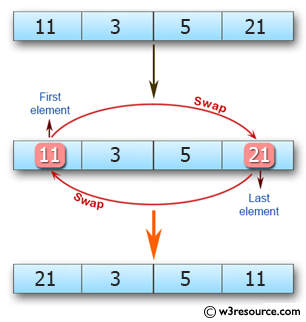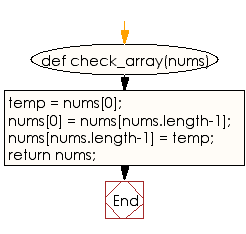﻿ Ruby Array exercises: Swap the first and last elements of a given array of integers - w3resource# Ruby Array Exercises: Swap the first and last elements of a given array of integers

## Ruby Array: Exercise-24 with Solution

Write a Ruby program to swap the first and last elements of a given array of integers, length will be at least 1. Return the modified array.Ruby Code:

``````def check_array(nums)
temp = nums;
nums = nums[nums.length-1];
nums[nums.length-1] = temp;
return nums;
end
print check_array(),"\n"
print check_array([1, 3]),"\n"
print check_array([1, 3, 5]),"\n"
print check_array([11, 3, 5, 21])
``````

Output:

```
[3, 1]
[5, 3, 1]
[21, 3, 5, 11]
```

Flowchart:-->

Ruby Code Editor: